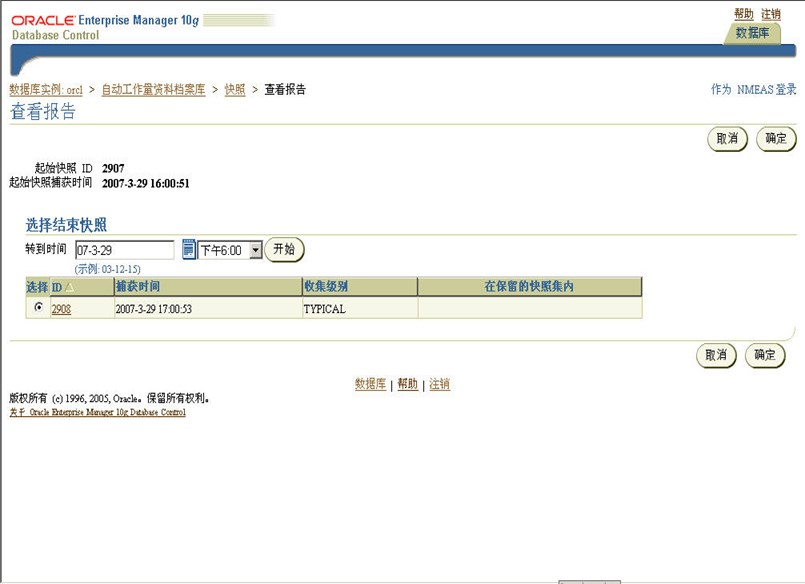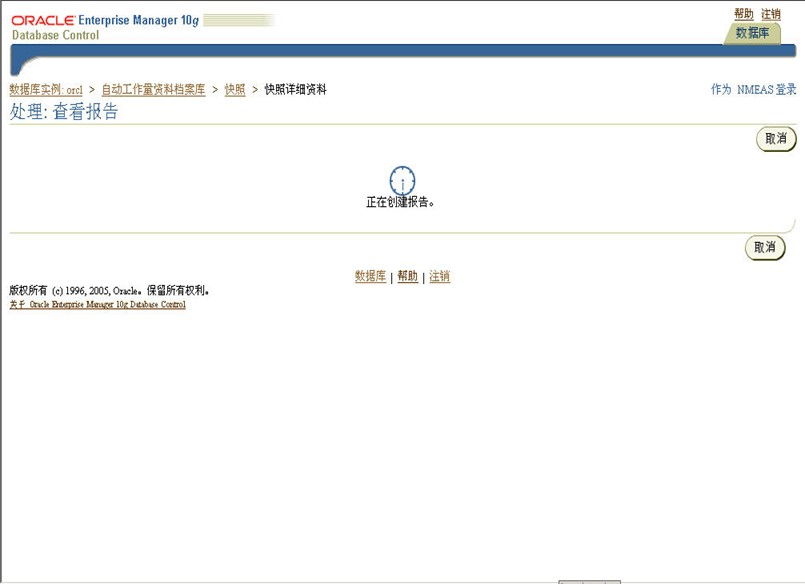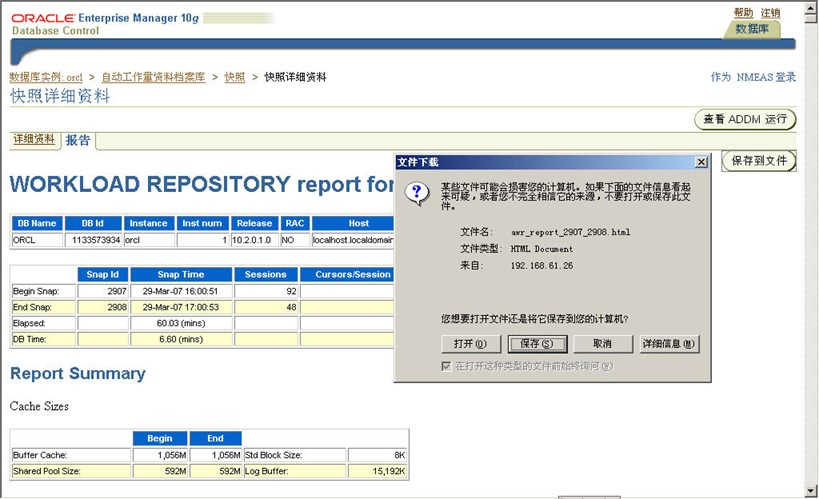# Oracle DBA日常维护工作手册参考5#### 3.2 每天工作结束后、系统空闲时运行

##### 3.2.1表空间使用率

###### 3.2.1.1 SQL脚本方式查看

 脚本：FREESPACE.SQL SELECT a.tablespace_name, ROUND (100 – b.free / a.total * 100) used_pct,        ROUND (a.total / 1024 / 1024) “total(MB)”,        ROUND (b.free / 1024 / 1024) “free_total(MB)”,        ROUND (b.max_free / 1024 / 1024) “free_max(MB)”, b.free_cnt fragment   FROM (SELECT   tablespace_name, SUM (BYTES) total             FROM dba_data_files         GROUP BY tablespace_name) a,        (SELECT   tablespace_name, SUM (BYTES) free, MAX (BYTES) max_free,                  COUNT (BYTES) free_cnt             FROM dba_free_space         GROUP BY tablespace_name) b WHERE a.tablespace_name=b.tablespace_name

###### 3.2.1.2 图形界面查看表空间使用率

Oracle 9i OEM 表空间管理